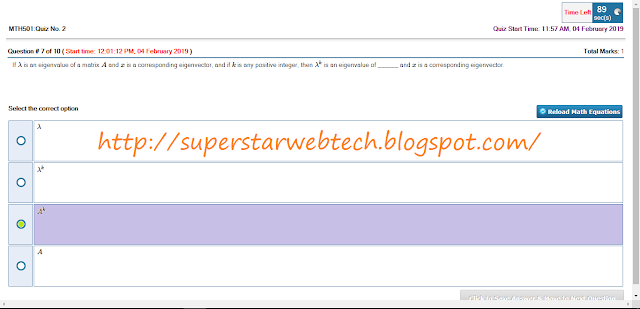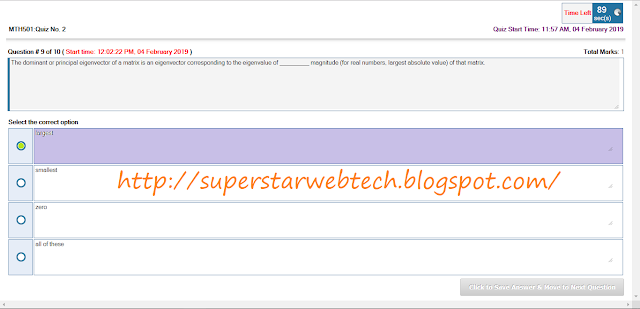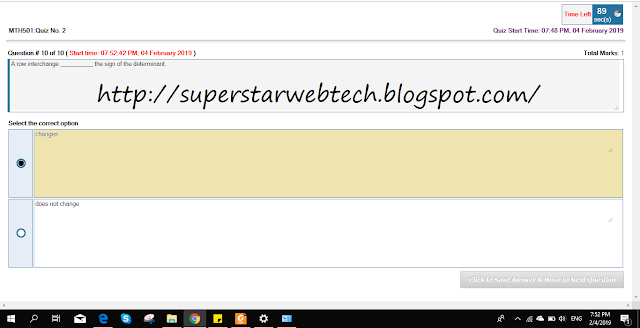# MTH501 Linear Algebra Quiz 2 Lecture 28-32 FALL 2018 Due date: 04 Feb, 2019 | SSWT

MTH501-Linear Algebra
QUIZ 2
Lecture 28-32
FALL 2018
due date: 04 Feb., 2019The null-space of a linear transformation T is the set of all vectors v such that T(v)=If A is an n x n matrix, then the following statements are equivalent EXCEPTIf A is triangular matrix then the eigenvalues of A are the entries on the main diagonal of AA row interchange ____ the sign of determinantThe characteristics polynomial of a 3 x 3 identity matrix is ___ if x is the eigen value of the given 3 x 3 identity matrixAn n x n matrix with n distinct eigen values is ___If   λ is an eigen value of a matrix A and x is a corresponding eigenvector, and if k is any positive integer then λ k is an eigenvalue of ___ and x is corresponding eigenvector.If 3 is an eigenvalue of A and x is a corresponding eigenvector then what is the eigenvalue of A^2?The dominant or principal eigenvector of a matrix is an eigenvector corresponding to the eigenvalue of ___ magnitude (for real numbers, largest absolute value) of that matrix.If n x n matrices A and B are similar. then they have the ___ characteristic polynomial.An n x n matrix A is diagonalizable if and only if A has n linearly ___ eigenvectorsIf A is a m x n matrix and A=A^T which of the following must always be true?An n x n matrix with n distinct values is ___The dominant or principal eigenvector of a matrix is an eigenvector corresponding to the eigenvalue of ___ magnitude (for real numbers, largest absolute value) of that matrixIf n x n matrices A and B are similar, then they have the ___ characteristic polynomialTwo matrices with the same characteristic polynomial ___ similarIf x-2 is a factor of the characteristic polynomial of matrix C then an eigenvalue of C isIf A and B are n x n matrices, then det (AB) =A row interchange ___ the sign of the determinant